# Pre-Algebra : Multiplication and Division

## Example Questions

← Previous 1 3 4

### Example Question #1 : Multiplication And Division

Express in degrees: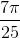radians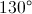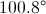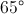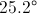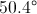Explanation:

Since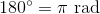, we can convert as follows: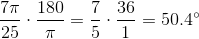### Example Question #1 : Multiplication And Division

Suppose you know the values ofand, and you want to evaluate the expression: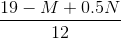In which order would you carry out the four operations in the expression?

Explanation:

A fraction bar in an expression acts as both a division symbol and a grouping symbol, so we evaluate the numerator first. Within the numerator, there is a multplication, a subtraction, and an addition, so, by order of operations, we multiply first. Addition and subtraction are carried out right to left; the subtraction is left of the addition, so we subtract next, then add. Finally, we divide the numerator by the denominator.

In summary: Multiply, subtract, add, divide

### Example Question #181 : Operations And Properties

Simplify the following as far as possible: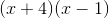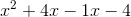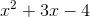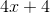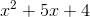Explanation:

If we use the foil method we get the following:
First: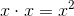Inner: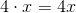Outer: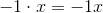Last: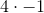If that is simplified we're left wiht### Example Question #1 : Multiplication And Division

Simplify: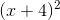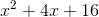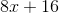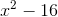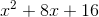Explanation:

The whole operation is squared, so to better understand how to solve it it can be rewritten as: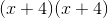Using order of operations we get the following:
First:Inner:Outer: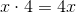Last: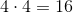When this is simplified we have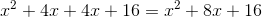### Example Question #1 : Multiplication And Division

Simplify: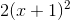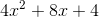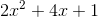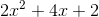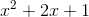Explanation:

The equation can be rewritten as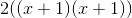First the foil method should be used, leaving us with: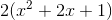Then the 2 can be distributed to each of the factors inside the parentheses. Leaving the solution:### Example Question #3 : Multiplication And Division

Which expression simplifies to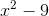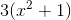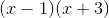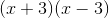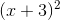Explanation:results inwhen simplifying with the foil method as follows:
First:Inner: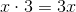Outer: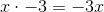Last: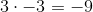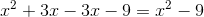### Example Question #5 : Multiplication And Division

What expression simplifies to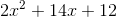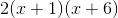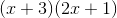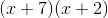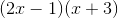Explanation:

When simplified using the FOIL methodresults in the following:

First:Inner: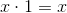Outer: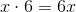Last: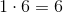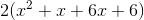When the 2 is distributed and the expression simplified we are left with### Example Question #1 : Multiplication And Division

A salesman for a car dealership earns 12% commission on all sales. What will be his commission for a week if he sells three cars that week - a $55,000 SUV on Tuesday, a$28,000 car on Thursday, and a $42,000 van on Friday? Possible Answers: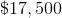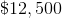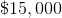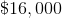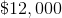Correct answer:Explanation: The total sale price of the cars he sells will be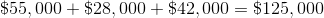. 12% of$125,000 is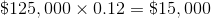,

which is the salesman's commission.

### Example Question #4 : How To Multiply

Read the following problem but do not solve it.

Acording to Mr. Smith's will, when he dies, each of his six children is to be left a parcel of land 510 acres in area. If these six parcels make up all of the farm, then how large is Mr. Smith's farm now?

Which of the following expressions must be evaluated in order to answer this question?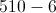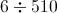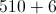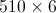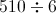Explanation:

There are six parcels of equal size; the total size is therefore the product of the common size (510 acres) and the number of parcels (6). This is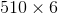### Example Question #7 : Multiplication And Division

Beck has 5 pairs of the same style of shoe, called the "button up." Each shoe has 6 buttons. How many buttons are there on Becky's "button up" shoe collection?Explanation:

If Beck has 5 pairs of shoes, that means he has 10 shoes because there are 2 shoes in a pair.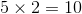Since each shoe has 6 buttons, that means that there are 60 buttons in the collection.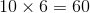← Previous 1 3 4

### All Pre-Algebra Resources